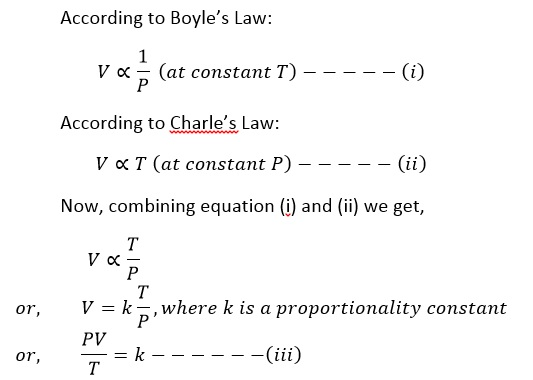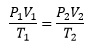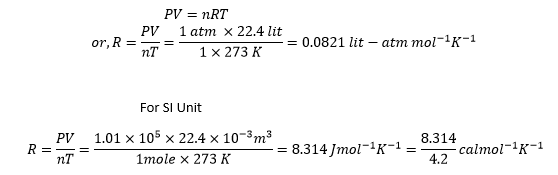# Combined Gas Equation

It studies the variation of the volume of a given mass of a gas with both temperature and pressure.If P1, V1, and T1 are the pressure, volume and temperature in the initial state respectively and P2, V2 and T2 are the pressure, volume and temperature in the final state respectively, thenThis equation is known as combined equation. Using this equation we can determine the state of the gas in any unknown condition if the initial state is known.
Now from equation (iii), we can write
PV=kT
The value of k depends on the amount of gas taken, for 1 gm of a gas the value of k depends upon the nature of gas and varies from one to another
For 1 mole of gas the value of k is constant called universal gas constant (R).
i.e. PV = RT
For n mole of gas the equation becomes
PV = nRT
This equation is called ideal gas equation.

Significance of universal gas constant:Therefore, Universal Gas Constant represents the work or energy required per mole of gas to increase its temperature by 1K.